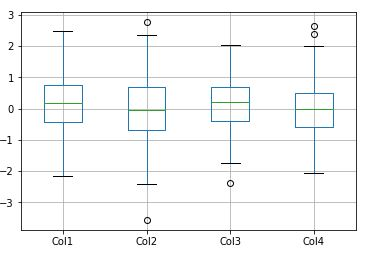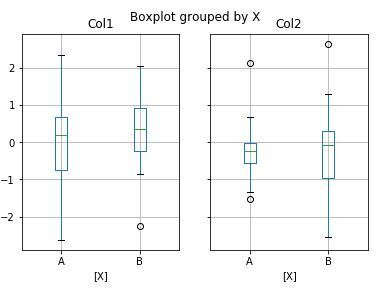# Pandas Statistical Functions Part 2 – std() , quantile() and boxplot()

## Introduction

In this article, we will learn about a few pandas statistical functions. The statistical functions that will be discussed in this article are pandas std() used for finding the standard deviation, quantile() used for finding intervals in the available data and finally the boxplot() function which is used to visualize the features that are used to describe the dataset. We will be looking at the syntax and examples of these functions to understand their usage.

### Importing Pandas Library

We will commence the tutorial by importing pandas library.

In :
import pandas as pd
import numpy as np

## Pandas Standard Deviation : std()

The pandas standard deviation functions helps in finding the standard deviation over the desired axis of Pandas Dataframes.

### Syntax

pandas.DataFrame.std(axis=None, skipna=None, level=None, ddof=1, numeric_only=None, kwargs)

• axis : {index (0), columns (1)} – This is the axis over which the standard deviation is calculated.
• skipna : bool, default True – It is used for deciding whether to exclude NA/Null values or not.
• level : int or level name, default None – This parameter is generally used when we have a multindex dataframe. Using this we can decide the level on which this function is applied.
• ddof : int, default 1 – Delta degrees of freedom or ddof is the divisor used in calculations is N – ddof, where N represents the number of elements.
• numeric_only : bool, default None – This parameter decides if only the int, float, boolean values should be included or not.
• kwargs – Additional Keyword Arguments

### Example 1: Applying pandas std() function over the rows

Here the standard deviation is computed using std() function of pandas.

In :
df = pd.read_csv('employees.csv')

In :
df.head()

Out:
First Name Gender Start Date Last Login Time Salary Bonus % Senior Management Team
0 Douglas Male 8/6/1993 12:42 PM 97308 6.945 True Marketing
1 Thomas Male 3/31/1996 6:53 AM 61933 4.170 True NaN
2 Maria Female 4/23/1993 11:17 AM 130590 11.858 False Finance
3 Jerry Male 3/4/2005 1:00 PM 138705 9.340 True Finance
4 Larry Male 1/24/1998 4:47 PM 101004 1.389 True Client Services

Here the skipna parameter is also used to decide whether to skip the null values or not. In this case, skipping the Null values did not made any difference.

NOTE: – The count of Null values present in a database can cause an alteration in the standard deviation value, in the example that we considered, the count of Null/NaN values are less and thus standard deviation is identical in both cases(Including and Excluding the Null Values).

Therefore, remember that a lesser number of Null Values or absence of Null values will have no effect on standard deviation. But a larger number of null values can cause a change in standard deviation.

Thus, an individual must analyze the dataset and then understand the impact of null values.

In :
df.std(axis=0,skipna=True)

Out:
Salary     32923.693342
Bonus %        5.528481
dtype: float64
In :
df.std(axis=0,skipna=False)

Out:
Salary     32923.693342
Bonus %        5.528481
dtype: float64

### Example 2: Applying pandas std() function over the columns

Using the pandas standard deviation function, we will be now computing the standard deviation over the columns. Again the standard deviation is the same for both cases of skipna.

In :
df.std(axis=1,skipna=True)

Out:
0       68802.235807
1       43790.295644
2       92332.689683
3       98072.641707
4       71419.631156
5       81425.378786
6       46291.444052
7       32452.242873
8       67565.097339
9       98884.977291
10      44707.440009
11      72475.166217
12      79754.225953
13      77658.121745
14      29282.322064
15      42011.154171
16      63896.029146
17      79005.455027
18      93989.282479
19      57284.209511
20      45756.219373
21      71133.778997
22      64203.304519
23      88944.810987
24      69251.299525
25      26203.555804
26      26580.315732
27      86522.845810
28      87677.377236
29      56230.539797
...
970     44940.597864
971     53328.319008
972     54001.632449
973     97119.627936
974     47828.380946
975     65353.306926
976     97545.242579
977     88077.256727
978     46764.381109
979    101060.975374
980     32685.401434
981    105334.670776
982     64631.229280
983    103870.635884
984     30718.104504
985     60575.073864
986     58585.918849
987     96660.555829
988     33677.226882
989     27110.619655
990     71243.849360
991     95101.583366
992     79731.504491
993     39902.714485
994     69911.308752
995     93667.850828
996     29961.758342
997     68527.541793
998     42771.485587
999     91880.628540
Length: 1000, dtype: float64

Again in this example, there is no difference in the standard deviation values of the dataframe calculated over columns. This suggests that count of null values is not causing any effects in our case.

In :
df.std(axis=1,skipna=False)

Out:
0       68802.235807
1       43790.295644
2       92332.689683
3       98072.641707
4       71419.631156
5       81425.378786
6       46291.444052
7       32452.242873
8       67565.097339
9       98884.977291
10      44707.440009
11      72475.166217
12      79754.225953
13      77658.121745
14      29282.322064
15      42011.154171
16      63896.029146
17      79005.455027
18      93989.282479
19      57284.209511
20      45756.219373
21      71133.778997
22      64203.304519
23      88944.810987
24      69251.299525
25      26203.555804
26      26580.315732
27      86522.845810
28      87677.377236
29      56230.539797
...
970     44940.597864
971     53328.319008
972     54001.632449
973     97119.627936
974     47828.380946
975     65353.306926
976     97545.242579
977     88077.256727
978     46764.381109
979    101060.975374
980     32685.401434
981    105334.670776
982     64631.229280
983    103870.635884
984     30718.104504
985     60575.073864
986     58585.918849
987     96660.555829
988     33677.226882
989     27110.619655
990     71243.849360
991     95101.583366
992     79731.504491
993     39902.714485
994     69911.308752
995     93667.850828
996     29961.758342
997     68527.541793
998     42771.485587
999     91880.628540
Length: 1000, dtype: float64

## Pandas Quantile : quantile()

The pandas quantile() function is used for returning values at the given quantile over requested axis.

### Syntax

pandas.DataFrame.quantile(q=0.5, axis=0, numeric_only=True, interpolation=’linear’)

• q : float or array-like, default 0.5 (50% quantile) – Here a value between 0 <= q <= 1 is specified, the quantile(s) to compute is executed using this information.
• axis : {0, 1, ‘index’, ‘columns’} (default 0) – This parameter is used to decide the axis over which this operation is performed.
• numeric_only : bool, default True – If False, the quantile of datetime and timedelta data will be computed as well.
• interpolation : {‘linear’, ‘lower’, ‘higher’, ‘midpoint’, ‘nearest’} – This parameter specifies the interpolation method to use.

### Example 1: Computing quantile using pandas quantile()

In this example, we will calculate different quantiles for the data.

NOTE: Quantiles are used to divide the data into fixed portions. The quantiles can range from 0% to 100%. Generally, quantiles that are frequently used are 25%, 50%, and 75%.

In :
df = pd.DataFrame(np.array([[5, 75], [10, 150], [15, 300], [20, 600]]),
columns=['P', 'Q'])

In :
df

Out:
P Q
0 5 75
1 10 150
2 15 300
3 20 600

In the below mentioned example, the 10% percentile is computed.

In :
df.quantile(.1)

Out:
P     6.5
Q    97.5
Name: 0.1, dtype: float64

In the below mentioned example, the 25%, 50% and 75% percentile are computed.

In :
df.quantile([.25, .5,.75])

Out:
P Q
0.25 8.75 131.25
0.50 12.50 225.00
0.75 16.25 375.00

### Example 2: Computing quantile of datetime and timedelta data

In this example, we will compute the quantile of datetime and timedelta data using pandas quantile function.

In :
df_date = pd.DataFrame({'P': [9, 27],
'Q': [pd.Timestamp('2017'),
pd.Timestamp('2019')],
'R': [pd.Timedelta('9 days'),
pd.Timedelta('25 days')]})

In :
df_date

Out:
P Q R
0 9 2017-01-01 9 days
1 27 2019-01-01 25 days

Here the numeric-only parameter of the quantile method is used, using this parameter we will be able to calculate the quantile of timedata. With the help of quantile function, we have calculated the 40% and 85% quantile of the data.

In :
df_date.quantile([0.40,0.85], numeric_only=False)

Out:
P Q R
0.40 16.2 2017-10-20 00:00:00 15 days 09:36:00
0.85 24.3 2018-09-13 12:00:00 22 days 14:24:00

## Pandas Boxplot : boxplot()

The pandas boxplot function helps in building a box plot from DataFrame columns.

### Syntax

pandas.DataFrame.boxplot(column=None, by=None, ax=None, fontsize=None, rot=0, grid=True, figsize=None, layout=None, return_type=None, backend=None, kwargs)

• column : str or list of str, optional – Here the column name or list of names is provided using this data, the boxplot is created.
• by : str or array-like, optional – This parameter is used for specifying the column used.
• ax : object of class matplotlib.axes.Axes, optional – Here the matplotlib axes to be used by boxplot is provided.
• fontsize : float or str – Here the tick label font size in points or as a string is given.
• rot : int or float, default 0 – The rotation angle of labels (in degrees) with respect to the screen coordinate system.
• grid : bool, default True – This is used for displaying the grid.
• figsize : A tuple (width, height) in inches – This is the size of the figure to create in matplotlib.
• layout : tuple (rows, columns), optional – The layout parameter helps in deciding the layout.
• return_type : {‘axes’, ‘dict’, ‘both’} or None, default ‘axes’ – This parameter helps in specifying the kind of object that should be returned.
• backend : str, default None – This is provided for the backend of the matplotlib plots.
• kwargs – Additional Keyword arguments.

The below image is used to explain the boxplot and it also helps in understanding the points represented by a boxplot. Here we can see that at two extreme ends, Outliers are represented, moving ahead the lower and upper limit of the dataset is represented in the form of whiskers or bars.

The two edges of the box represent the minimum and maximum value in the range of the dataset. The middle section is displaying the median of the dataset. The Q1, Q2 and Q3 are the quartiles which represent the 25%, 50% and 75% intervals of the dataset respectively.

The difference between Q3 and Q1 quartiles is known as the Interquartile range.

NOTE: Boxplot is a very important visualizing tool to learn for data science aspirants.

### Example 1: Visualizing data through pandas boxplot() function

Here the data is generated using numpy and then boxplot function is used to visualize the data using pandas boxplot function.

In :
np.random.seed(1234)

In :
df = pd.DataFrame(np.random.randn(100, 4),
columns=['Col1', 'Col2', 'Col3', 'Col4'])

In :
df.head()

Out:
Col1 Col2 Col3 Col4
0 0.471435 -1.190976 1.432707 -0.312652
1 -0.720589 0.887163 0.859588 -0.636524
2 0.015696 -2.242685 1.150036 0.991946
3 0.953324 -2.021255 -0.334077 0.002118
4 0.405453 0.289092 1.321158 -1.546906

In this example, we can see how all the descriptive statistics information is provided. In the 4 columns information which are conveyed in the below visualization, Col1 doesn’t have outliers, but other three do have outliers.

Outliers are basically those values which do not belong to the range of data to which other data belongs to.

Apart from this, we can see that the minimum values and maximum values are falling almost similar for all the 4 columns.

This is how data is explained with the help of boxplot. Here we are able to decipher the minimum and maximum values, median value, outliers, quartiles, starting and ending values as well.

In :
boxplot = df.boxplot(column=['Col1', 'Col2', 'Col3','Col4'])### Example 2: Grouped Boxplots by a variable

Here the boxplot is created by grouping them with another variable which consists series data. We can see how the series data is visualized using the boxplot using these two columns.

There are outliers as well in the data which can be easily seen along with other details of the dataset.

In :
df = pd.DataFrame(np.random.randn(50, 2),
columns=['Col1', 'Col2'])

In :
df['X'] = pd.Series(['A', 'A', 'A', 'A', 'A','A', 'A', 'A', 'A', 'A','A', 'A', 'A', 'A', 'A','A', 'A', 'A', 'A', 'A',
'B', 'B', 'B', 'B', 'B','B', 'B', 'B', 'B', 'B','B', 'B', 'B', 'B', 'B','B', 'B', 'B', 'B', 'B'])

In :
boxplot = df.boxplot(by='X')## Conclusion

We have reached to the finish point of this tutorial, in this tutorial we covered some of the most important concepts of statistical pandas functions with focus being on pandas functions like std(), quantiles() and boxplot() which is used to describe a dataset efficiently. We looked at the syntax and examples of these functions to better understand them and their usage.

Reference – https://pandas.pydata.org/docs/